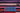# Crawler Log Folder - minimum number of operations needed to go back to the main folder after the change folder operations.

October 03, 2020## Problem

The Leetcode file system keeps a log each time some user performs a change folder operation.

The operations are described below:

”../” : Move to the parent folder of the current folder. (If you are already in the main folder, remain in the same folder). “./” : Remain in the same folder. “x/” : Move to the child folder named x (This folder is guaranteed to always exist). You are given a list of strings logs where logs[i] is the operation performed by the user at the ith step.

The file system starts in the main folder, then the operations in logs are performed.

Return the minimum number of operations needed to go back to the main folder after the change folder operations.

Example

``````Input: logs = ["d1/","d2/","../","d21/","./"]
Output: 2
Explanation: Use this change folder operation "../" 2 times and go back to the main folder.

Input: logs = ["d1/","d2/","./","d3/","../","d31/"]
Output: 3

Input: logs = ["d1/","../","../","../"]
Output: 0``````

## Solution

This is fairly simple problem. The only complexity in this problem is that for `../`. If you are in the main folder, and you do a `../`, you should remain in the same folder.

If you see, the problem is asking you to calculate how far you have gone, or the distance. Because, that is how many steps you will require to come back to original main folder.

Lets try to calculate the distance from the main folder.

• Keep an integer variable for calculating distance
• If the string is `./`, Just keep the counter as it is
• If its `../`, you need to check if you are in the current folder or not. If you are in the current folder, your `distance` counter will be zero. So if its zero, do nothing else decrement the counter
• Now the third operation is changing to a folder, i.e. `abc/`, just increment the counter.

Lets look at the code

``````public int minOperations(String[] logs) {
int distance = 0;
for (int i=0; i<logs.length; i++) {
if (logs[i].equals("./")) {
continue;
}
else if (logs[i].equals("../")) {
if (distance == 0) {
//do nothing
continue;
}
else {
distance --;
}
}
else {
// folder/
distance ++;
}
}

return distance;
}``````

### Complexity

Its simple `O(n)` where n is the length of array

## Leetcode Submission Result

Your runtime beats 100% of java submissions. Memory usage beats 80% of java submissions.